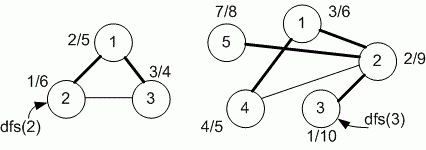favorite We need a little bit of your help to keep things running, click on this banner to learn more
Competitions

# Depth First Search - Timestamps

Undirected graph is given. Run depth first search from the given vertex v. Print the timestamps d[v] and f[v] for each vertex v in the increasing order of vertices.

#### Input

First line contains number of vertices n (n100) and edges m of undirected graph. Each of the next m lines contains two vertices a and b - an undirected edge of the graph. The last line contains vertex v.

#### Output

Run dfs(v). Print the timestamps d[v] and f[v] for each vertex v (v = 1, 2, ..., n). The timestamps for each vertex must be printed in a separate line.Time limit 1 second
Memory limit 128 MiB
Input example #1
```3 3
1 2
2 3
1 3
2
```
Output example #1
```2 5
1 6
3 4
```
Input example #2
```5 5
1 2
2 3
2 5
2 4
1 4
3
```
Output example #2
```3 6
2 9
1 10
4 5
7 8
```